Function Repository Resource:

# ImageROIConvert

Simple tool for working with regions of interest on images

Contributed by: Michael Sollami
 ResourceFunction["ImageROIConvert"][roi] represents a rectangular region of interest roi, given by<|"x"→xmin,"y"→ymin,"w"→width,"h"→height|>. ResourceFunction["ImageROIConvert"][roi,image,transform] represents a rectangular region of interest roi in an image after applying a coordinate system transform. ResourceFunction["ImageROIConvert"][roi, {width,height}, transform] represents a rectangular region of interest roi in an image of given dimensions after applying a coordinate system transform.

## Details and Options

ResourceFunction["ImageROIConvert"] is a simple helper function when working with rectangles in systems other than the Wolfram Language. Many imaging and machine learning libraries assume a coordinate system whose origin is placed at the top left corner of an image with the positive y axis going down vertically (i.e. "TopLeft"), as opposed to the Wolfram Language convention of a bottom left corner origin with a positive y axis going up (i.e. "BottomLeft").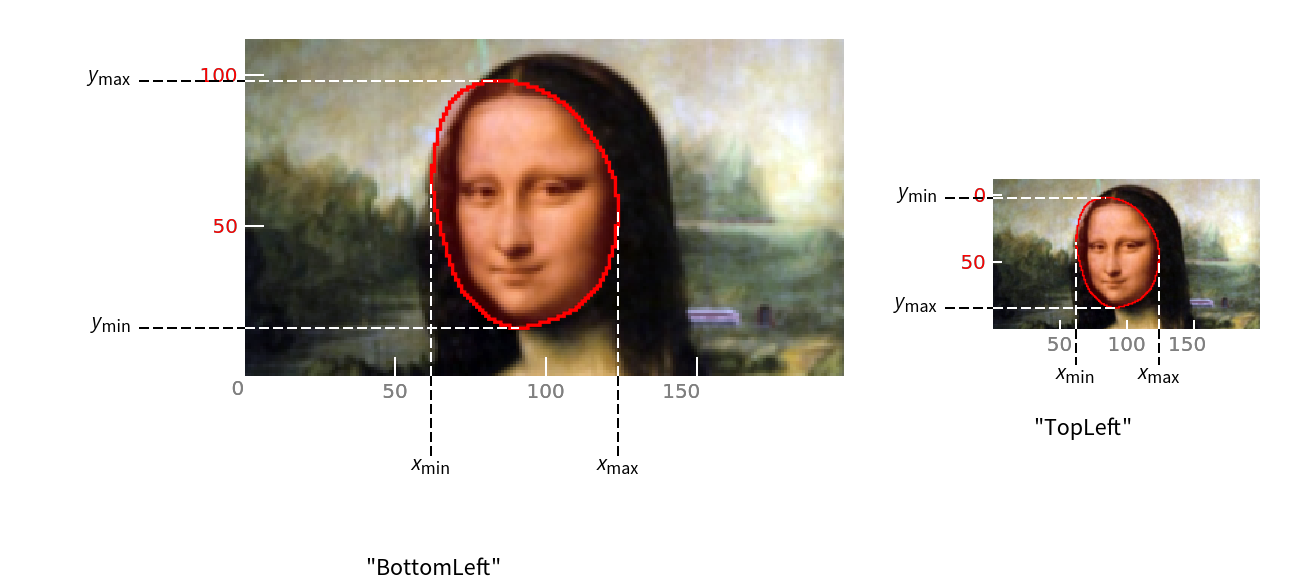The first argument roi may be a Rectangle or an Association with keys "x","y","w","h", and both may be given with scaled or unscaled values depending on the option "ScaledCoordinates".
If the optional third argument is not provided, the input roi is assumed to be given in the normal "BottomRight" coordinate system (the default for both Graphics and Image), and no transformation is performed.
ResourceFunction["ImageROIConvert"] takes a boolean-valued option "ScaledCoordinates" which indicates the roi was given in scaled coordinates (defaults to False).
ResourceFunction["ImageROIConvert"] always returns a Rectangle object in unscaled coordinates.
The examples were tested with Python 3.8.5 and OpenCV version 4.4.0 (both installed with anaconda). Make sure to have libraries cv2 and zmq installed and configured for the external evaluator.

## Examples

### Basic Examples (4)

Sometimes ROIs (regions of interest) are provided in terms of width and height:

 In:=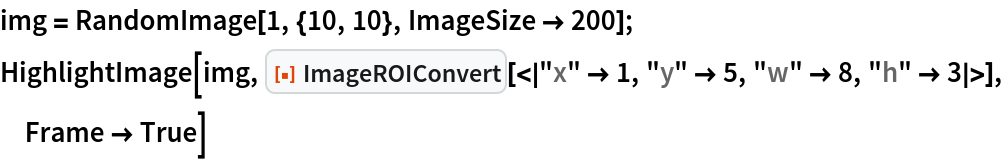Out=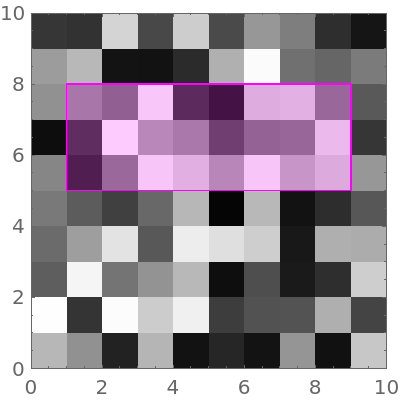Having to switch between coordinate systems with a top or bottom origin is a very common. When converting to a "TopLeft" or "BottomRight" origin, you need to supply the original image (or its dimensions):

 In:=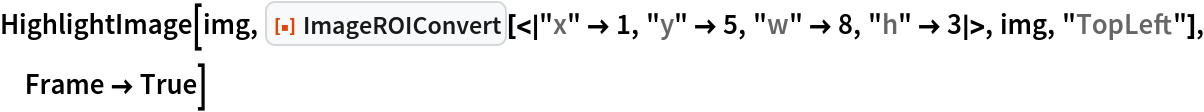Out=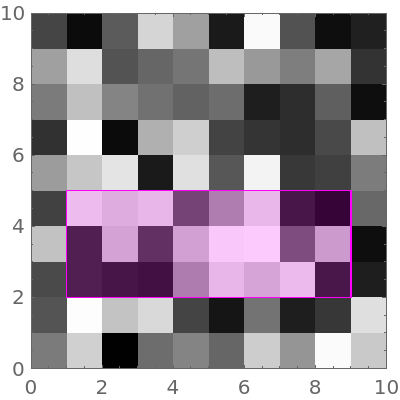As a convenience, ImageROIConvert handles regions given in XYWH form. They can be given as real pixel coordinate values in the range [0,w][0,h] or as scaled coordinates in the range [0,1]⨯[0,1]:

 In:=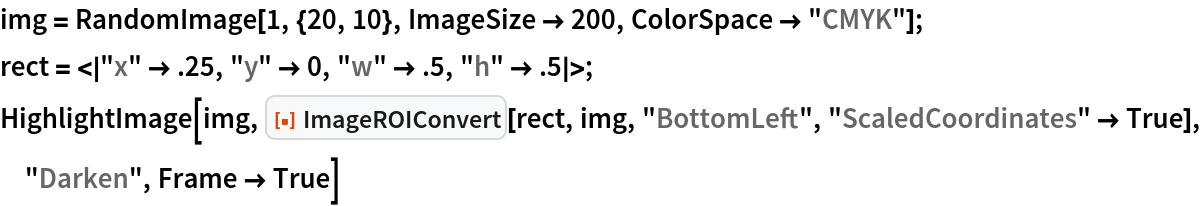Out=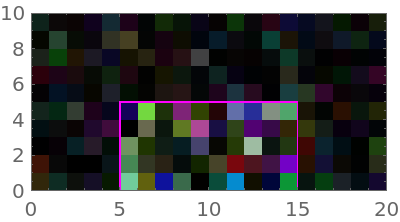To run this example, you first need to connect to a python executable in an environment (e.g. here my environment is named dl) where both opencv and pyzmq are installed (for more details see this workflow):

 In:=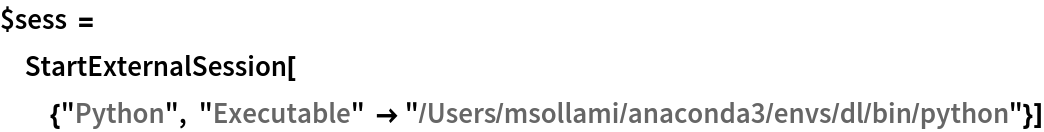Out=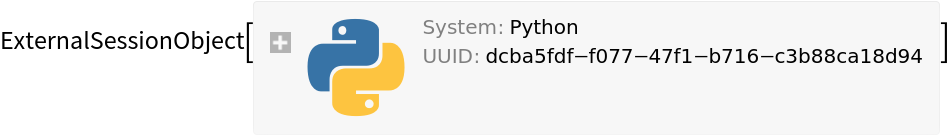Now let's draw a rectangle on an image with the python computer vision library OpenCV (which is named cv2 in code):

 In:=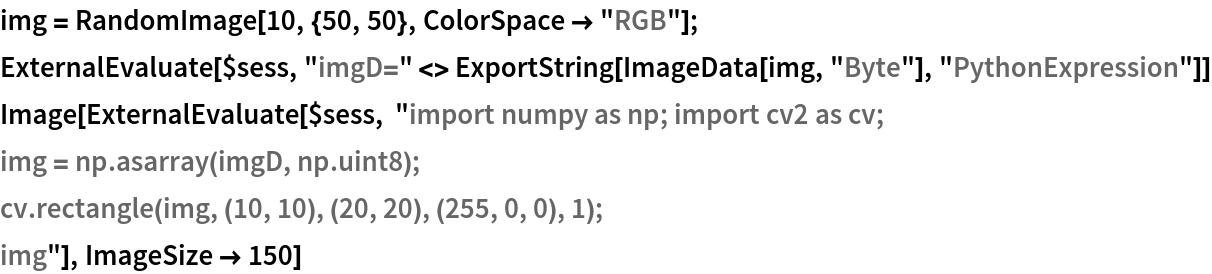Out=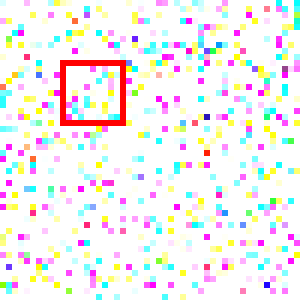Convert the coordinate system's origin to "BottomLeft" to plot it:

 In:=Out=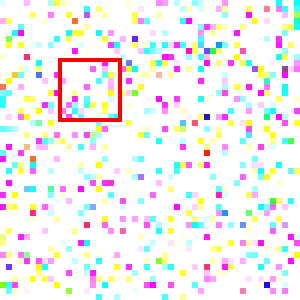Create a Rectangle region of interest and highlight it:

 In:=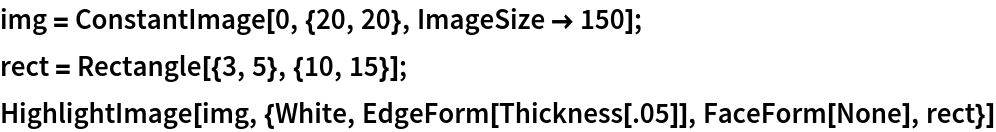Out=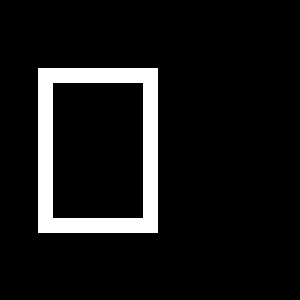To use this in OpenCV (whose python library is named cv2), we ask for a "TopLeft" origin coordinate transform:

 In:=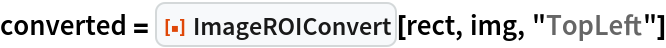Out=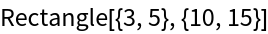Now we can send it to a session for drawing (requires a python installation):

 In:=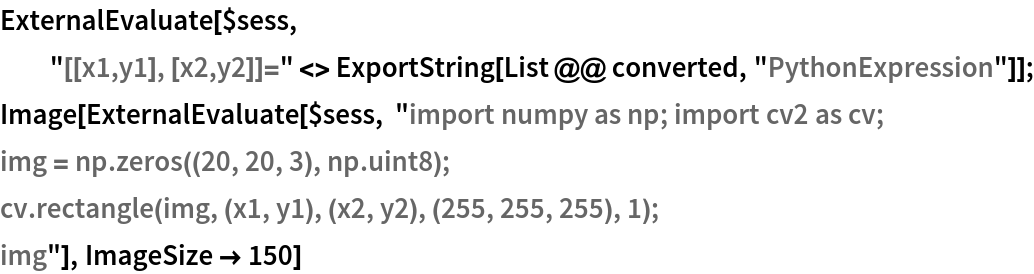Out=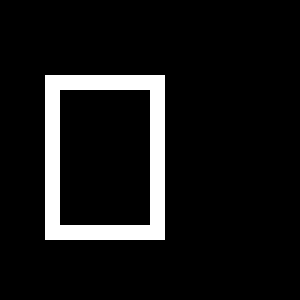### Options (2)

Create a Rectangle with scaled coordinates for use in OpenCV (this example requires a python installation):

 In:=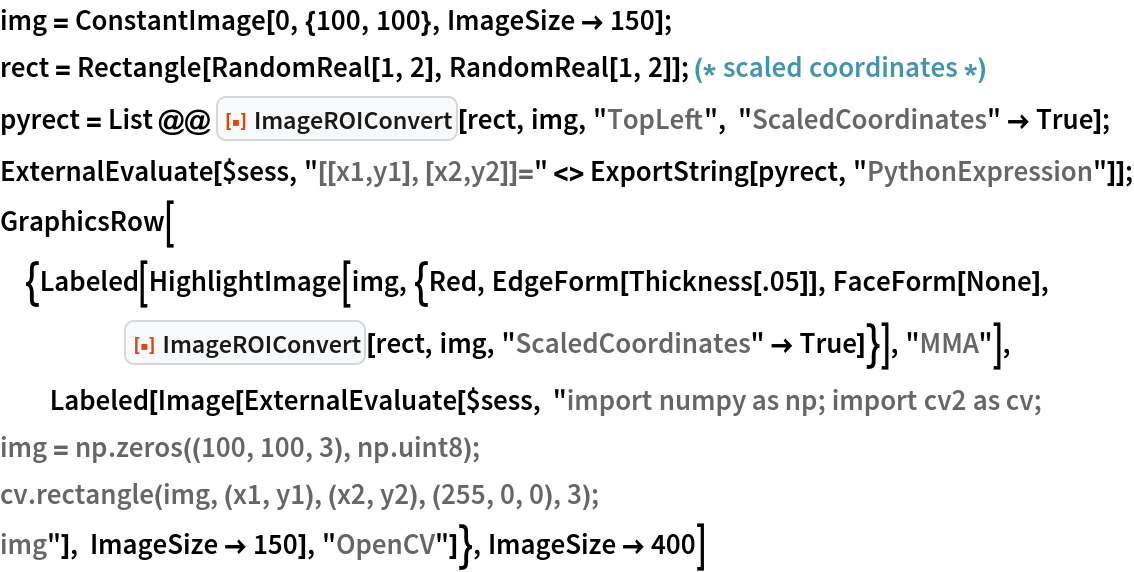Out=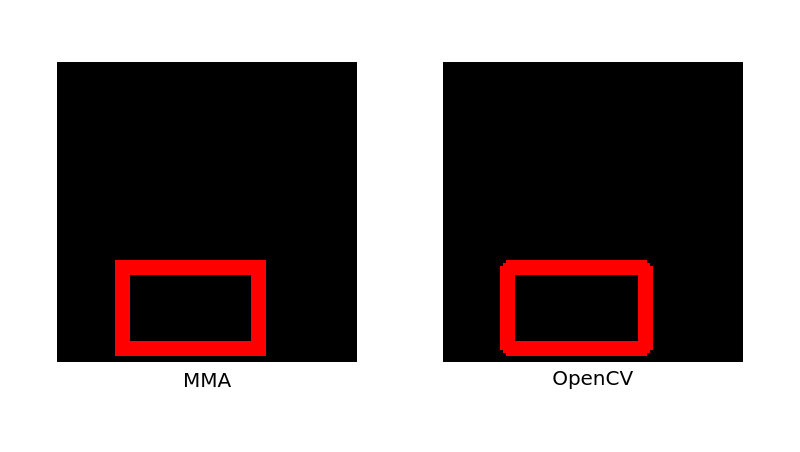If setting the option "ScaledCoordinates"True, the coordinates of the input are assumed to lie between zero and one:

 In:=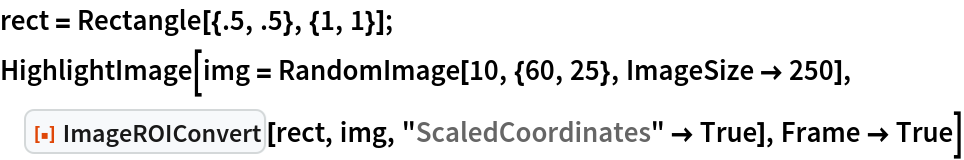Out=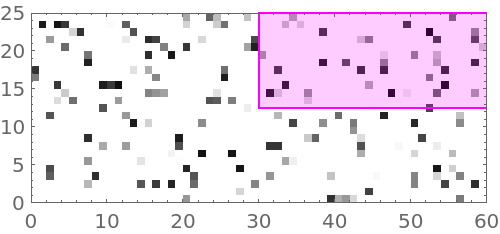In:=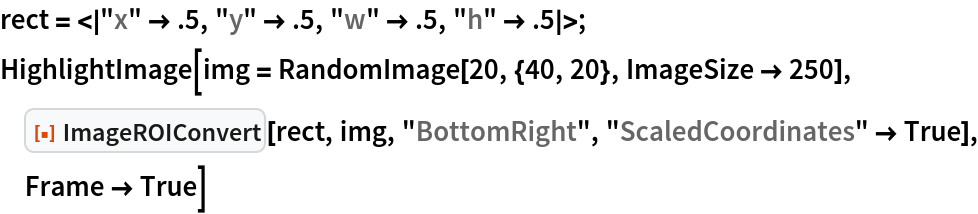Out=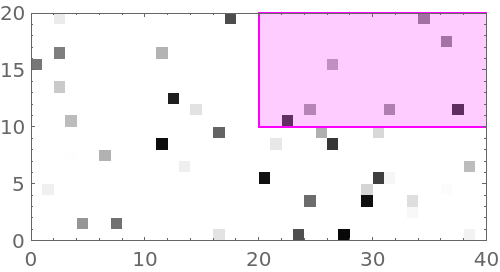### Applications (5)

Convert object detections from the Wolfram Language to Python (this example requires a python installation):

 In:=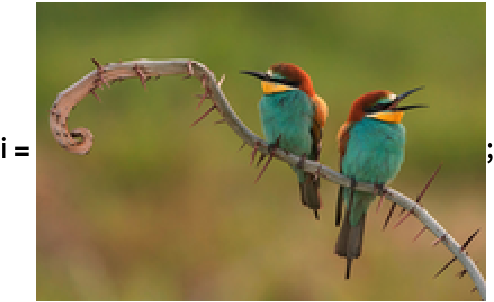In:=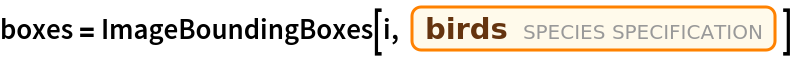Out=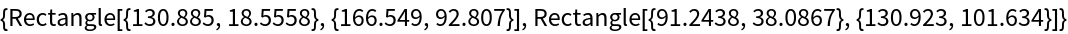Highlight the identified bounding boxes:

 In:=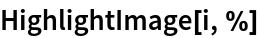Out=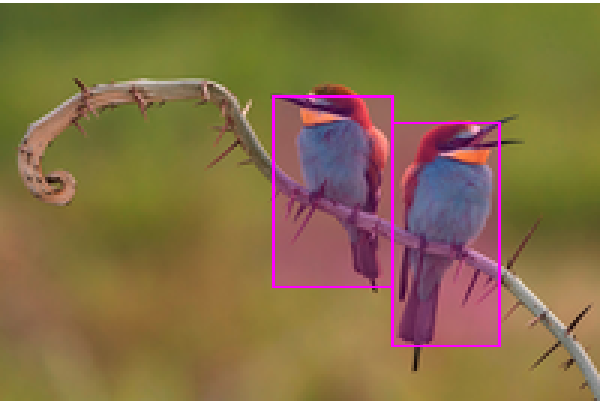Convert the bird's bounding boxes to have top left origin:

 In:=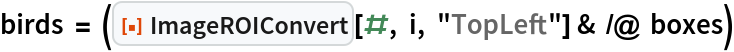Out=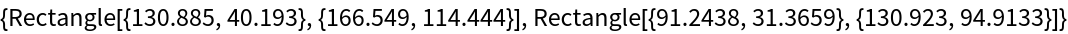Export the variables to the python session:

 In:=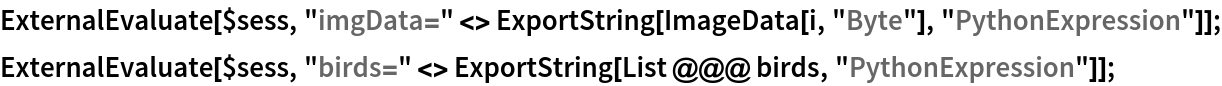Draw the boxes:

 In:=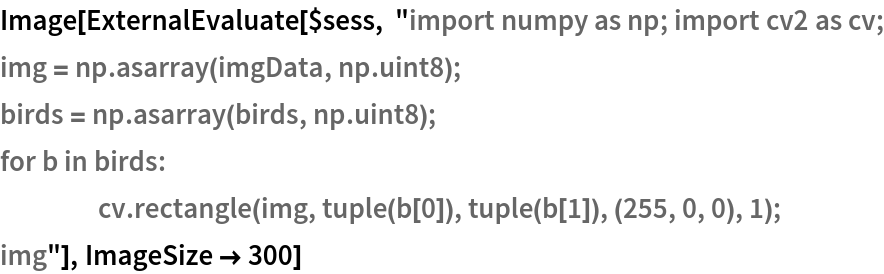Out=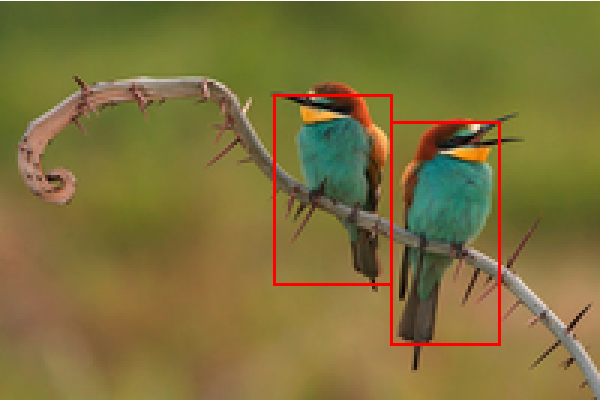Michael Sollami

## Version History

• 1.0.0 – 23 December 2020